# Novel Image Steganography Based on Preprocessing of Secrete Messages to Attain Enhanced Data Security and Improved Payload Capacity

Novel Image Steganography Based on Preprocessing of Secrete Messages to Attain Enhanced Data Security and Improved Payload Capacity

Department of Electrical Engineering, International Islamic University, Islamabad 30001, Pakistan

Department of Electrical Engineering, Air University, Islamabad 30001, Pakistan

Corresponding Author Email:
laeeq.msee424@iiu.edu.pk
Page:
129-136
|
DOI:
https://doi.org/10.18280/ts.370117
23 November 2019
|
Accepted:
10 January 2020
|
Published:
29 February 2020
| Citation

OPEN ACCESS

Abstract:

Image steganography hides secret messages in cover images by manipulating their content. There is a fundamental requirement of maximizing secrecy and payload capacity. This paper presents a novel algorithm for achieving two layer security as well improved payload capacity by prepossessing the secret images before hiding it in a cover image. The secret image is divided in to small windows of ﬁxed sizes and is searched from a database of 255 images. Each window of secret image is replaced by the address of its closet match in database. Instead of hiding a secret image this algorithm hides the address of database. Consequently makes it impossible for any third party to retrieve the secret image without having access to the database. The size of the database address is less than the size of secret message thus it improves the payload capacity of the proposed algorithm.

Keywords:

data security, hidden communication, Steganography

1. Introduction

Steganography is an art of secret communication using public channel. The word Steganography has been derived from two Greek words “steganos” means covered and “graphein” mean writing [1, 2]. The history of steganography can be traced back in Greek times where they use to tatoo secret messages on slaves shaved heads and the messages were sent when their hair grew back. The receiver simply shaves their heads again and the messages were retrieved. They also used wax tablets for same purpose . The use of invisible inks in ﬁrst World War and the use of microdots in Second World War were also among conventional types of steganography techniques.

The evolution in the technology and the exponential growth in multimedia communications has generated concern regarding information security. Encryption schemes like RSA  and data encryption standard (DES)  are the most widely used solution for securing information. These schemes basically scramble data such that it becomes unreadable, however, such data is more suspicious and attains more attraction from unintended users. Thus, there is always a chance that one might keep on trying and somehow manages to decrypt the message. On the other hand, Stenography hides the existence of information with the help of a cover medium such that a secret message is embedded into a cover medium to generate the stego-output as shown in Figure 1(a). On the receiving side a retrieving algorithm regenerates the secret message out of the stego-message as shown in Figure 1(b). Another type of data hiding schemes having similar principals are known as watermarking and are used in copyright protection.

steganography can be classiﬁed into text [6-8], image [9, 10], audio [11, 12], video [13, 14] and network or protocol Steganography  techniques. Text Steganography hides secret information with the help of text, whereas, image Steganography hides data within an image and same is for the others. The ﬁgures of merit or the comparison parameter for comparing diﬀerent embedding algorithms are payload capacity, imperceptibility, robustness against channel impairments and data security . In Image Steganography payload capacity is measured in bits per pixel that is the maximum number of bits that can be hidden within a single pixel on average. The imperceptibility is the measure of diﬀerence between the stego-image and the host or the cover image. The subjective test for imperceptibility asks a group of people to select the original image (cover image) from a set cover and stego-images. If the rate of success is below 50 percent it is concluded that the said embedding algorithm is imperceptible. The rules and recommendations for subjective tests are discussed by Rodrigues et al.  beside the subjective test, the peak signal to noise ratio (PSNR) is calculated from the error matrix between the host and the stego-images. An image has high correlation among adjacent pixels and this property makes them ideal for data embedding. The main idea is to ﬁnd out that location where the correlation among adjacent pixel is low and updates their pixel values with the help of secret data. The boundary pixel in an image has the least correlation and as the human visual system is less sensitive against changes in such places, thus they are ideal for data hiding. Steganography schemes on the basis of secret image retrieval are divided in to lossless and lossy schemes. In lossless Steganography schemes the recovered secret message is exactly similar to the one embedded by the sender, whereas, in lossy schemes, the estimate of secret image is retrieved at the receiver side.

In this paper, novel stenography algorithm based on pre-processing of secret data is proposed to attain enhanced two layer data security and maximum payload capacity. The main idea is to regenerate a secret image from ﬁnite number of indexed images already existing in the data set. The transmitter and the receiver have already agreed on the said data-set. Instead of embedding pixel intensity values directly into the host image, the proposed scheme embeds their indexes into the host image. Receiver retrieves these indexes from the host and then using the indexed data set re-generates an estimate of the secret message. The results have shown that the quality of recovered signal was above 45 dB in term of PSNR that mean the recovered image and the original secrete image was close enough. Furthermore, the rate of success was below 50 percent in the subjective test for ﬁnding out the host-images among from set of host and stego-images.

## 1.jpg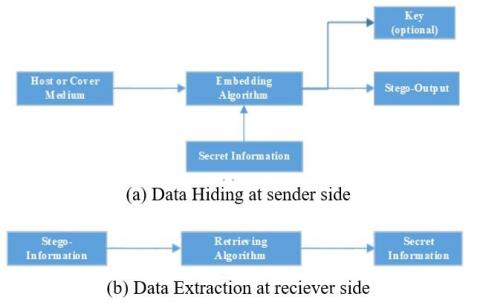Figure 1. Basic steganography block diagram

2. Literature Review

The fundamental requirements for any Steganography algorithms are high capacity data embedding, less distortion in the host image and high data security. Quality of the stego-image and the high capacity data embedding are inversely related to each other i.e. increasing one results in decreasing other. As imperceptibility is the lifeline of every Steganography algorithm, therefore, one cannot improve capacity at the cost of decreasing quality of stego-images below a certain level. Hence capacities of various image Steganography algorithms [18-21] were very less during the past few years. Recently proposed algorithms [22, 23] attained signiﬁcant improvement in term of capacity as well as image quality, however, it requires a secret key to be sent along with the secret message for recovering the secret image from the host image. Once this secret key is disclosed the communication will no more be secure.

The image Steganography algorithms can be further classiﬁed into two main categories that are spatial domain techniques and frequency domain techniques. Spatial domain techniques hide secret information directly into the pixel intensity value as in the study , the authors proposed a scheme that substitute adaptively chosen number of bits. The said adaptive number of substituting bits was relatively high in boundary pixel values. Method presented by Yang et al. , use LSB substitution and pixel value diﬀerencing (PVD) to hide in edges areas of an image. Chang et al.  used dynamic programming strategy to ﬁnd out number of optimal substitution bits. Beside such substitution techniques Chung et al.  proposed a Steganography method based on singular value decomposition. Another scheme  propose an algorithm for reversible data hiding scheme for the images compressed with block truncation codes.

Frequency domain techniques transforms images to its frequency domain equivalent using Discrete Fourier Transform (FFT) [21, 28], Discrete Cosine Transform (DCT) [22, 23, 29-32] or Discrete Wavelet Transform (DWT) [33, 34]. Later on they substitute bits of the frequency domain coeﬃcients. As already discussed, human visual system is not sensitive against changes in high frequencies or low correlating areas therefore, more bits can be replaced in place of coeﬃcients representing higher frequency. Rabie  proposed that the changes in the Fourier magnitude are not obvious if the Fourier Phase are maintained. Hence they substitute the Fourier magnitudes with secret image while maintaining the phase of those Fourier magnitudes to achieve higher imperceptibility. Liu and Qiu  proposed an algorithm that hides data after taking 4th level DWT of the host image using Mallet algorithm with bi-orthogonal 9/7 basis. They used HL4.1, LH4.2, HH4.3, HL3.1, LH3.2, HH3.3, HL2.1, LH2.2, and HH2.3 for hiding message. These sub-bands have high energy of all high frequency sub-bands. The algorithm proposed by Rabie and Kamel , ﬁrst converts image into N ×N block size and later on take DCT of each block. Later on they quantize each block with the standard Jpeg Quantization matrix with quality factor 50 to calculate an average block size for data embedding. The results achieved in the study  were improved by Rabie and Kamel  by replacing adaptive number of DCT coeﬃcients in each block of N ×N size. The size of data embedded in each block is sent as an adaptive key along with stego-image for retrieving secret image. This scheme achieved high capacity as well as acceptable PSNR then all existing schemes. However, sending key along with stego-image reduces security while communicating i.e. in case the key is decoded then the secret data can easily be extracted from each block.

The work presented in this paper presents enhanced two layer data security with improved payload capacity and an acceptable level of peak signal to noise ratio. The main idea is to convert secret message in to small blocks and then ﬁnd those small block with in a large but ﬁnite indexed data set of images. The index of the block that is highly similar to the secret image block is stored. This reduces the size of secret image to be sent or subsequently increases payload capacity. Later on, these indexes are embedded in to the host image DCT coeﬃcients. This makes the communication imperceptible. Moreover, embedding indexes into the host image make this problem unsolvable for an unintended receiver to regenerate secret image from indexes until and unless they get the same data set indexed in same order. Thus this scheme achieved better payload capacity and enhanced data security as compared to the Steganography schemes [19, 21-23, 29] that have been recently published in the literature. One of the limitations of proposed scheme is its computational time for generating estimate of the secret images. Therefore, this scheme cannot work for real time online applications. However, this scheme is fit for offline use where sender requires two layer security at the cost of spending time before sending messages on channel.

3. Mathematical Concepts

This section explains the mathematical concepts related to the work presented in this paper.

3.1 Secret image estimation

This section explains the basic concept behind image estimation from a given dataset of image. Suppose we have a data set (D) of n images such that images {I1, I2..., In} has unique image number. Each image of size m×m is further sub divided into non-overlapping window size of x1×k. So, the data set is given as

$D=\left(\begin{array}{l}I_{11} I_{21} I_{31} \ldots . I_{n 1} \\ I_{12} I_{22} I_{32} \ldots . . I_{n 2} \\ I_{13} I_{23} I_{33} \ldots . . I_{n 3} \\ \ldots \ldots \ldots \ldots \ldots \ldots \\ \ldots \ldots \ldots \ldots \ldots \ldots \\ I_{1 p} I_{2 p} I_{3 p} \ldots . . I_{n p}\end{array}\right)$

In $I_{i j}$ i represent the image number and j represents the window number and p is the total number of non over lapping windows in each image given by

$p=\frac{m \times m}{k}$    (1)

Now if we divide and rearrange secret image (SI) of size m×m in to non-overlapping window size of 1×k we have

$SI={{\left( S{{I}_{1}}S{{I}_{1}}S{{I}_{1}}....S{{I}_{P}} \right)}^{T}}$

where, SI1 represents ﬁrst window of size 1×k and p the total number of windows is given by Eq. (1). Deﬁne another matrix R having p rows and two columns where each row represents corresponding window of secret image i.e. ﬁrst row of matrix R will save the index of that data-set image and window within that image that is very close to secret image window SI1.

Now we start matching secret image windows with data set images one by one and calculate error matrix EI1 = [e1,e2,e3,......ep] deﬁne as

$E_{11}=\frac{1}{p}\left(\begin{array}{l}\sum\left|S I_{1}-S I_{11}\right| \sum\left|S I_{2}-S I_{11}\right| \ldots . \sum\left|S I_{p}-S I_{11}\right| \\ \sum\left|S I_{1}-S I_{12}\right| \sum\left|S I_{2}-S I_{12}\right| \ldots . \sum\left|S I_{p}-S I_{12}\right| \\ \sum\left|S I_{1}-S I_{13}\right| \sum\left|S I_{2}-S I_{13}\right| \ldots . \sum\left|S I_{p}-S I_{13}\right| \\ \dot\\ \\ \dot\\ \\ \sum\left|S I_{1}-S I_{1 p}\right| \sum\left|S I_{1}-S I_{1 p}\right| \ldots . \sum\left|S I_{p}-S I_{1 p}\right|\end{array} |\right.$

If minimum (e1) is less below a speciﬁed threshold then the index of corresponding data set image is stored at the ﬁrst row of matrix R. Calculate EI2 for the remaining windows of Secret image which had error above acceptable level with ﬁrst data set image. This iterative process continuous till all Windows ﬁnd their equivalent window in the data set. R is a matrix of size p×2 where ﬁrst column represents the image number and second column represents the window number with in that speciﬁc image and p is the total number of windows given by Eq. (1). The secret image can be regenerated from these indexes if we have data set D. The regenerated secret image SI0 is an estimate of actual secret image.

3.2 Discrete cosine transform

2D-Discrete cosine transform converts an image from spatial to its frequency domain equivalent. Due to its high energy compaction capability it is widely used in signal processing specially for lossy data compression. The Jpeg image compression standard  ﬁrst divides an image into window size of 8×8 and later takes 2DDCT of the image using

$F(u, v)$

$=\frac{2}{N} c(u) c(v) \sum_{x=0}^{N-1} \sum_{y=0}^{N-1} F(x, y)$

$\times\left\{\cos \frac{\pi u(2 x+1)}{2 N} \cos \frac{\pi v(2 y+1)}{2 N}\right.$    (2)

This returns 8×8 window of DCT coeﬃcients where F(0,0) represents DC coeﬃcient and F(7,7) represents highest frequency coeﬃcient. Human visual system is not sensitive to the changes in high frequency coeﬃcients. Hence these coeﬃcient are heavily quantized using Jpeg standard quantization matrix. Most Steganography schemes using DCT hides information in these high frequency components to make the changes imperceptible. Authors [22, 23] calculate a square block of zero coeﬃcients in the lower right corner of each window after quantization and embeds secret image pixel value in place of these zero coeﬃcients. This results in improving capacity as well as better imperceptibility. Inverse DCT transform is applied on the stego-image DCT coeﬃcients using Eq. (3) to take stego-image back to spatial domain.

$F^{\prime}(x, y)$

$=\frac{2}{N} \sum_{u=0}^{N-1} \sum_{v=0}^{N-1} c(u) c(v) D(x, y)$

$\times\left\{\cos \frac{\pi u(2 x+1)}{2 N} \cos \frac{\pi v(2 y+1)}{2 N}\right.$    (3)

3.3 Image quality measurement

Beside subjective tests that totally based upon perceptions of human visual system peak signal to noise ratio is used for calculating diﬀerence between two images. Recently published schemes [19-23, 29] have used peak signal to noise ratio as ﬁgure of merit for comparing host and the stego images. This paper compares host image with stego image and use their diﬀerence matrix to calculate peak signal to noise ratio denoted as PSNRhs. As the retrieved image is an estimate of original secrete image therefore, it also calculates diﬀerence between embedded and the retrieved secret message and is denoted by PSNRss0. Peak signal to noise ratio is calculated using

$P S N R=20 \log _{10} \frac{\operatorname{Max}_{X}}{\sqrt{M S E}}$    (4)

where,

$M S E=\frac{1}{M \times N} \sum_{i=0}^{M} \sum_{j=0}^{N}\left[F(i, j)-F^{\prime}(i, j)\right\}^{2}$    (5)

4. Proposed Algorithm

Proposed algorithm further more consist of two main algorithms i.e. embedding algorithm and retrieving algorithm. Both algorithms are discussed as following.

4.1 Embedding algorithm

Step 1: Rearrange the given data set of images as matrix D and the secret image as matrix SI.

Step 2: Starting from the ﬁrst column of matrix D i.e. image I1 calculate error matrix for ﬁrst data set image i.e. EI1. If any element of EI1 is less than a speciﬁc threshold (i.e. 2 per pixel on average in each window) store the corresponding index of data set image in matrix R. Calculate EI2 for the renaming windows of secret image and so on until all windows ﬁnd their equivalent.

In case after calculating x (initially x=5) consecutive error matrix we cannot ﬁnd equivalent of even single secret image window we increase error threshold level and at the same time also increase x by one i.e. if we were increasing threshold after ﬁve unsuccessful searches next time we will increase on x+1 unsuccessful searches. There might be few windows that could not ﬁnd their equivalent in the whole data set. These windows are left blank and are estimated by the average of the neighboring pixels on the receiver side

Step 3: Third step is to divide the host image in to non-overlapping window size of 8×8. Then take DCT of each window using (2) and quantize with the standard Jpeg quantization matrix to calculate a non-zero coeﬃcients block with in lower right corner of each window.

Step 4: Re-scale all elements of matrix R in the range [0, 10] using

$R^{\prime}=\frac{R}{255} \times 10$    (6)

Later embed all these values of matrix R in place of zero DCT coeﬃcients. Size of zero coeﬃcients block is already calculated in the previous step and is sent as a secret key with the stego-image.

Step 5: Finally take Inverse discrete transform to generate stego-image.

4.2 Retrieving algorithm

Step 1: Divide stego-image into non-overlapping window of size 8 × 8 and take 2D-DCT using (2).

Step 2: Retrieve data out of each window using the key received along with the stego-image and take re-scale in range [0,255] using

$R=\frac{R^{\prime}}{10} \times 255$    (7)

Step 3: Each Row in matrix R represents corresponding window of embedded secret image. Now we start regenerating secret image by the coping windows from the indexes speciﬁed my matrix R.

Step 4: Rearrange data to generate secret image estimate.

5. Results and Comparisons

This section compares results of the proposed algorithm with the recently published algorithms. The proposed algorithm is test on ﬁve diﬀerent Host images with three diﬀerent secret images. Data set of 143 images is used to estimate secret images. Peak signal to noise ratio is used to measure quality of the stego-image and the recovered image.

5.1 Results of the proposed algorithm

Five gray scale host images of size 1024×1024 are shown in Figure 2 (from left to right) Baboon, Barbara, Boat, Jet and Peppers. Whereas, gray scale secret images each of size 512×512 are shown in Figure 3 (from left to right) Tiger face, Tomatoes and Balloons. These three secret images were ﬁrst estimated using data set of 143 images of same size. Total number of bits in each secret image before estimation is given by 512×512×8=2097152. Each secret image is divided into non over lapping windows of size 1×4 and thus we have total number of windows given as 512×512 1×4=65536. These windows are matched with non-overlapping windows of data set images and a matrix R is generated. Where matrix R contains 65536 rows and 2 columns, where each row represents corresponding window of secret image. First column of matrix R represent image number of data set and as we have use 143 images so it requires only 8 bits for representation whereas, the second column represents the window with in each image. Each Image in data set could have 65536 windows at maximum and if we start indexing from 0 we require 16 bit to represent window with in image. Therefore total number of bits after estimation can be given by 24×65536=15728462. Therefore, 524288 less bits are required to represent a secret image of size 512×512. Moreover, this gives us high data security where one can never recover the secret message until and unless he has the same data set arranged in same order as with the sender and receiver. The processor of the system used for estimation of matrix R is “core(TM) i7-4610M CPU @ 3.00 GHZ 3.00 GHZ”. Whereas, installed memory of the system is 8GHZ. The proposed algorithm takes 1086 seconds to estimate balloon image, 1530 seconds for tomatoes image estimation and 1230 seconds for the estimation of tiger face secret image.

These matrices R for three secret images are then embedded into ﬁve diﬀerent host images to generate ﬁfteen stego-images. PSNRHS is calculated between the host and the stego-images. This gives us imperceptibility and additional data security with high payload capacity. Table 1 shows result of the proposed algorithm using ﬁve diﬀerent host images and three diﬀerent secret images to generate 15 stego images are shown in Figure 4. These stego-images are sent to the receiver along with the key where receiver ﬁrst retrieves the matrix R and then regenerates the secret image. PSNRSS0 shows the quality of the recovered secret image over the error matrix between original secret and the recovered secret image.

## 2.jpg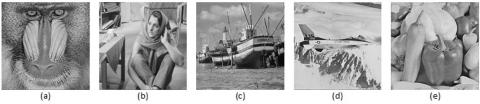Figure 2. Host Images that are used as cover images for data tramission

## 3.jpg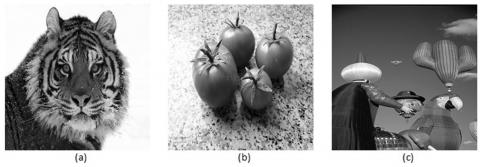Figure 3. Secret Images for hiding them in Host images

## 4.jpg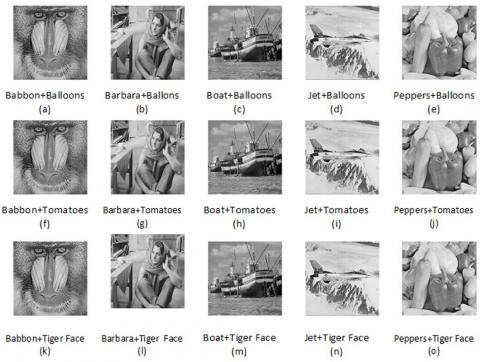Figure 4. Stego images with balloons secrete image from a to e and with tomatoes secret image from f to j and with tiger face as secret image from K to o

Table 1. Results comparison in term of payload capacity and PSNR

 Host Image Secret Image Estimation time (Sec) PSNRHS PSNRSS’ SI Bits Capacity Barbara Boat Jet Peppers Baboon Balloons Balloons Balloons Balloons Balloons 1086 1086 1086 1086 1086 36.37 37.06 36.30 37.50 36.00 46.27 46.27 46.27 46.27 46.27 6022110 5442260 6202710 5127280 5602340 5.74 5.19 5.92 4.89 5.34 Barbara Boat Jet Peppers Baboon Tomatoes Tomatoes Tomatoes Tomatoes Tomatoes 1530 1530 1530 1530 1530 36.60 37.00 36.20 37.40 36.40 48.25 48.25 48.25 48.25 48.25 6022110 5442260 6202710 5127280 5602340 5.74 5.19 5.92 4.89 5.34 Barbara Boat Jet Peppers Baboon Tiger face Tiger face Tiger face Tiger face Tiger face 1230 1230 1230 1230 1230 36.40 37.10 36.30 37.60 36.50 47.12 47.12 47.12 47.12 47.12 6022110 5442260 6202710 5127280 5602340 5.74 5.19 5.92 4.89 5.34 Average 1282 36.71 47.21 5679340 5.42

5.2 Comparison

In this section we compare the proposed algorithm results with the schemes recently published in the literature. Lee & Chen  proposed a high capacity model for high capacity data embedding and achieved capacity of 4.025 bits per pixel that mean in a host image of size 1024×1024 they can hide 4220519 bits with stego image quality of 32.57 db.

Another scheme proposed by Rabie , achieved capacity of 2 bits per pixel with 19.41 db PSNR which mean for a host image of size 1024×1024 they can hide 2097152 bits. Whereas, the schemes proposed in integer achieved capacity of 3.69 bits per pixel or 3869425 bits for selected host image size and 36.4 db PSNR. The scheme proposed by Rabie and Kamel  achieved capacity upto 2.72 bits per pixel i.e. 2852126 bits in 1024×1024 host image with 28.5 db PSNR using window size of 8×8. This scheme was improved by Rabie and Kamel  for achieving capacity of 3.07 bits per pixel on average i.e. 3219129 bits for host image size of 1024×1024 with 36.4 db PSNR. However, the proposed algorithm has achieved Average embedding capacity of 5.42 bits per pixel with 36.71 db PSNR between Host and stego images with window size of 8×8. Therefore, proposed algorithm has signiﬁcant improvement in term of payload capacity as well as stego image quality. However, existing schemes the recovered secret image was exactly similar to the embedded secret image whereas, the proposed algorithm recovers an estimate of the secret images. However, the quality of recovered image is very high and is above 45 db in term of PSNR. Figure 6 and Figure 7 shows the comparison of the proposed algorithm with recently published algorithms whereas Figure 5 shows the recover secret images.

## 5.jpgFigure 5. Recovered images at receiver end

## 6.jpg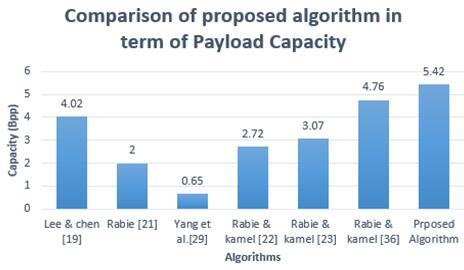Figure 6. Comparison in term of payload capacity

## 7.jpg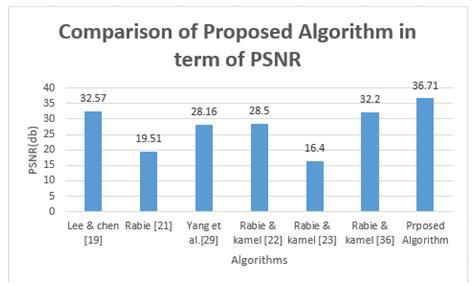Figure 7. Comparison in term of PSNR

6. Conclusion

This paper presents a Novel image Steganography scheme that is based on preprocessing of secret data for reducing its payload and increasing data security. The two layer data security is achieved by ﬁrst converting image into the index matrix of data set and then by embedding this matrix into the host image. Representing an image into an index matrix form reduces the size of image by 25 percent that means we can send 25 percent more data in the same host. Embedding indexes data into a host image makes indexes imperceptible. However, even if some third party manages to decode the indexes using the key sent along with the stego-image yet they cannot retrieve secret messages until and unless they have similar data-set of images ordered in similar order.

References

 Swanson, M.D., Kobayashi, M., Tewfik, A.H. (1998). Multimedia data-embedding and watermarking technologies. Proceeding of the IEEE, 86(6): 1064-1087. https://doi.org/10.1109/5.687830

 Johnson, N.F., Jajodia, S. (1998). Exploring steganography: Seeing the unseen. Computer (Long. Beach. Calif)., 31(2): 26-34. https://doi.org/10.1109/MC.1998.4655281

 Krenn, R. (2004). Steganography and steganalysis. Retrieved Sept., 8(2007): 2.

 Rivest, R.L., Shamir, A., Adleman, L. (1978). A method for obtaining digital signatures and public-key cryptosystems. Communications of the ACM, 21(2): 120-126. https://doi.org/10.1145/359340.359342

 Mahajan, P., Sachdeva, A. (2013). A study of encryption algorithms AES, DES and RSA for security. Global Journal of Computer Science and Technology, 13(15).

 Shirali-Shahreza, M. (2008). Text steganography by changing words spelling. Advanced Communication Technology, 2008. ICACT 2008. 10th International Conference on, 2008, 3: 1912-1913. https://doi.org/10.1109/ICACT.2008.4494159

 Shirali-Shahreza, M., Shirali-Shahreza, M.H. (2007). Text steganography in SMS. ICCIT '07: Proceedings of the 2007 International Conference on Convergence Information, pp. 2260-2265. https://doi.org/10.1109/ICCIT.2007.369

 Shirali-Shahreza, M.H., Shirali-Shahreza, M. (2006). A new approach to Persian/Arabic text steganography. 5th IEEE/ACIS International Conference on Computer and Information Science and 1st IEEE/ACIS International Workshop on Component-Based Software Engineering,Software Architecture and Reuse (ICIS-COMSAR'06), Honolulu, HI, pp. 310-315. https://doi.org/10.1109/ICIS-COMSAR.2006.10

 Nikolaidis, N., Pitas, I. (1998). Robust image watermarking in the spatial domain. Signal Processing, 66(3): 385-403. https://doi.org/10.1016/S0165-1684(98)00017-6

 Takano, S., Tanaka, K., Sugimura, T. (2000). Data hiding via steganographic image transformation. IEICE Trans. Fundam. Electron. Commun. Comput. Sci., 83(2): 311-319.

 Gopalan, K. (2003). Audio steganography using bit modification. Proceedings of the IEEE International Conference on Acoustics, Speech, and Signal Processing, (ICASSP'03), 2: II-421. https://doi.org/10.1109/ICASSP.2003.1202390.

 Sridevi, R., Damodaram, A., Narasimham, S.V.L. (2009). Efficient method of audio steganography by modified lsb algorithm and strong encryption key with enhanced security. Journal of Theoretical & Applied Information Technology, 5(6).

 Hu, S.D., Kin, T.U. (2011). A novel video steganography based on non-uniform rectangular partition. 2011 14th IEEE International Conference on Computational Science and Engineering, Dalian, pp. 57-61. https://doi.org/10.1109/CSE.2011.24

 Cao, Y., Zhao, X., Feng, D., Sheng, R. (2011). Video steganography with perturbed motion estimation. International Workshop on Information Hiding, pp. 193-207. https://doi.org/10.1007/978-3-642-24178-9_14

 Lucena, N.B., Pease, J., Yadollahpour, P., Chapin, S.J. (2004). Syntax and semantics-preserving application-layer protocol steganography. International Workshop on Information Hiding, pp. 164-179. https://doi.org/10.1007/978-3-540-30114-1_12

 Chen, B., Wornell, G.W. (2001). Quantization index modulation: A class of provably good methods for digital watermarking and information embedding. IEEE Transactions on Information Theory, 47(4): 1423-1443. https://doi.org/10.1109/18.923725

 Rodrigues, J.M., Rios, J.R., Puech, W. (2004). SSB-4 System of Steganography using bit 4. 5th International Workshop on Image Analysis for Multimedia Interactive Services.

 Brisbane, G., Safavi-Naini, R., Ogunbona, P. (2005). High-capacity steganography using a shared colour palette. IEE Proceedings-Vision, Image Signal Process., 152(6): 787-792. https://doi.org/10.1049/ip-vis:20045047

 Lee, Y.K., Chen, L.H. (2000). High capacity image steganographic model. IEE Proceedings-Vision, Image Signal Process., 147(3): 288-294. https://doi.org/10.1049/ip-vis:20000341

 Lin, C., Shiu, P.F. (2010). High capacity data hiding scheme for DCT-based images. Journal of Information Hiding and Multimedia Signal Processig, 1(3): 220-240.

 Rabie, T. (2013). High-capacity steganography. Image and Signal Processing (CISP), 2013 6th International Congress on, 2: 858-863.

 Rabie, T., Kamel, I. (2016). On the embedding limits of the discrete cosine transform. Multimedia Tools and Application, 75(10): 5939-5957. https://doi.org/10.1007/s11042-015-2557-x

 Rabie, T., Kamel, I. (2016). High-capacity steganography: A global-adaptive-region discrete cosine transform approach. Multimedia Tools and Applications, 76: 6473-6493. https://doi.org/10.1007/s11042-016-3301-x

 Yang, C.H., Weng, C.Y., Wang, S.J., Sun, H.M. (2008). Adaptive data hiding in edge areas of images with spatial LSB domain systems. IEEE Transactions on Information Forensics and Security, 3(3): 488-497. https://doi.org/10.1109/TIFS.2008.926097

 Chang, C.C., Hsiao, J.Y., Chan, C.S. (2003). Finding optimal least-significant-bit substitution in image hiding by dynamic programming strategy. Pattern Recognition, 36(7): 1583-1595. https://doi.org/10.1016/S0031-3203(02)00289-3

 Chung, K.L., Shen, C.H., Chang, L.C. (2001). A novel SVD-and VQ-based image hiding scheme. Pattern Recognition Letters, 22(9) 1051-1058. https://doi.org/10.1016/S0167-8655(01)00044-7

 Sun, W., Lu, Z.M., Wen, Y.C., Yu, F.X., Shen, R.J. (2013). High performance reversible data hiding for block truncation coding compressed images. Signal Image and Video Processing, 7(2): 297-306. https://doi.org/10.1007/s11760-011-0238-4

 Rabie, T. (2012). Digital image steganography: An FFT approach. Communications in Computer and Information Science, 294: 217-230. https://doi.org/10.1007/978-3-642-30567-2_18

 Yang, B., Schmucker, M., Funk, W., Busch, C., Sun, S. (2004). Integer DCT-based reversible watermarking for images using companding technique. Proc. SPIE, Secur. Steganography, Watermarking Multimed. Contents, pp. 405-415.

 Chang, C., Lin, C., Tseng, C.S., Tai, W.L. (2007). Reversible hiding in DCT-based compressed images. Information Science, 177(13): 2768-2786. https://doi.org/10.1016/j.ins.2007.02.019

 Iwata, M., Miyake, K., Shiozaki, A. (2004). Digital steganography utilizing features of JPEG images. IEICE Transactions on Fundamentals of Electronics Communications and Computer Sciences, 87(4): 929-936.

 Lin, C.C., Shiu, P.F. (2009). A DCT-based reversible data hiding scheme. Proceedings of the 3rd International Conference on Ubiquitous Information Management and Communication, pp. 327-335. https://doi.org/10.1145/1516241.1516298

 Sarreshtedari, S., Ghaemmaghami, S. (2010). High capacity image steganography in wavelet domain. Consumer Communications and Networking Conference (CCNC), 2010 7th IEEE, pp. 1-5. https://doi.org/10.1109/CCNC.2010.5421800

 Liu, T., Qiu, Z. (2002). A DWT-based color image steganography scheme. Signal Processing, 2002 6th International Conference on, 2: 1568-1571. https://doi.org/10.1109/ICOSP.2002.1180096

 Wallace, G.K. (1992). The JPEG still picture compression standard. IEEE Trans. Consum. Electron., 34(4). https://doi.org/10.1145/103085.103089